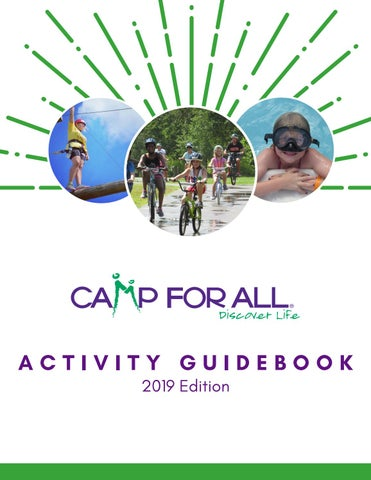X X

X X

X

X

X

X X

X X

X X X X X X X

X X

X X X X X X X X X X X X X X X X X X X X X X X X X X

X

X X X

X X X

X X

X X X X X X X X X X

X X X X

X X

30 minutes 1 hour

n/a 6 years and up 8 years and up 10 years and up 12 years and up 13 years and up MINIMUM TIME

Indoor Outdoor MINIMUM AGE

Animal Related Challenge Course Creative Arts Outdoor Experiences Sports Target Sports Water Activities Recharge Activities LOCATION

CATEGORY Advanced Archery Archery Arts & Crafts Barnyard BB Guns Beauty Spa Bikes Canoes Cast Fishing Cooking Dance Drop Fishing Escape Room Giant Swing Gold Panning High Ropes Horseback Riding Innertube Water Polo Kayaks Low Ropes/Teambuilding Mad Science Minute to Win It Nature Nerf Wars Outdoor Cooking Outpost Overdrive Race Cars Paintball Photography Recording Studio Scavenger Hunt Star Place Ropes Superhero Party Swimming Tea Party Topshot Tower Virtual DJ Wii Sports

X X X X X X X X X X X X X X X X X X X X X X X X X X X X X X X X X X X

X X X X X X X X X

X X

X X X X X X

X X X X X X X

X X X X X

X X X X

X X

X X X X X X X X X X X

X X X X X X X X X X X

X